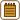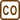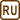## Determination of the Specific Heat Capacity

### Task number: 1794

To determine the specific heat capacity of ethanol, we have poured 160 g of water at a temperature of 15.0 °C into 200 g of ethanol at a temperature of 29.9 °C in a calorimeter with a heat capacity of 180 JK-1. The temperature stabilized at 22.4 °C. Using the specific heat capacity of water, determine the specific heat capacity of ethanol and compare it with the table value.

• #### Hint

To solve this problem, we will use the calorimetry formula, which states that the heat received by colder body from the warmer one equals the heat supplied by the warmer body to the colder one (the law of conservation of energy).

In this case the heat supplied by the ethanol and calorimeter is received by the water.

• #### Notation

 meth = 200 g = 0.200 kg mass of ethanol teth = tc = 29.9 °C temperature of ethanol in the calorimeter C = 180 JK−1 heat capacity of the calorimeter mw = 160 g = 0.160 kg mass of water tw = 15.0 °C temperature of water cw = 4180 Jkg−1K−1 specific heat capacity of water t = 22.4 °C resultant temperature ceth = ? specific heat capacity of ethanol
• #### Analysis

The specific heat capacity of ethanol can be determined by using the calorimetry formula, which states that the heat received by colder body from the warmer one equals the heat transferred from the warmer body to the colder one.

At the beginning we have warmed ethanol in the calorimeter and we assume thermal equilibrium, i.e. the calorimeter has the same temperature as the ethanol in it. Next, we pour colder water into this system. Ethanol and the calorimeter reduce their temperature, i.e. transfer the heat to the water, which receives it and therefore is being heated.

• #### Solution

The calorimetry formula:

$Q_{\mathrm{supplied}}=Q_{\mathrm{received}}.$

The heat supplied by the ethanol and calorimeter is received by the water. Then:

$c_{\mathrm{eth}} m_{\mathrm{eth}} (t_{\mathrm{eth}} - t) + C (t_c - t) = c_w m_w (t- t_w).$

Hence we can express the unknown specific heat capacity of ethanol ceth:

$c_{\mathrm{eth}} m_{\mathrm{eth}} (t_{\mathrm{eth}} - t) = c_w m_w (t- t_w) - C (t_c - t),$

$c_{\mathrm{eth}}=\frac{ c_w m_w (t- t_w) - C (t_c - t)}{ m_{\mathrm{eth}} (t_{\mathrm{eth}} - t)}.$

Substitution of the given values leads to:

$c_{\mathrm{eth}}=\frac{ 4180 \cdot{0.16} \cdot (22.4- 15.0) - 180\cdot (29.9 - 22.4)}{0.2 \cdot (29.9 - 22.4)}\,\mathrm Jkg^{-1}k^{-1},$

$c_{\mathrm{eth}}\dot=2400\,\mathrm Jkg^{-1}k^{-1}=2.4\,\mathrm kJkg^{-1} k^{-1}$

• #### Answer

Using the given values, we compute that the specific heat capacity of ethanol is 2.40 kJkg−1K−1, which matches well the table value.